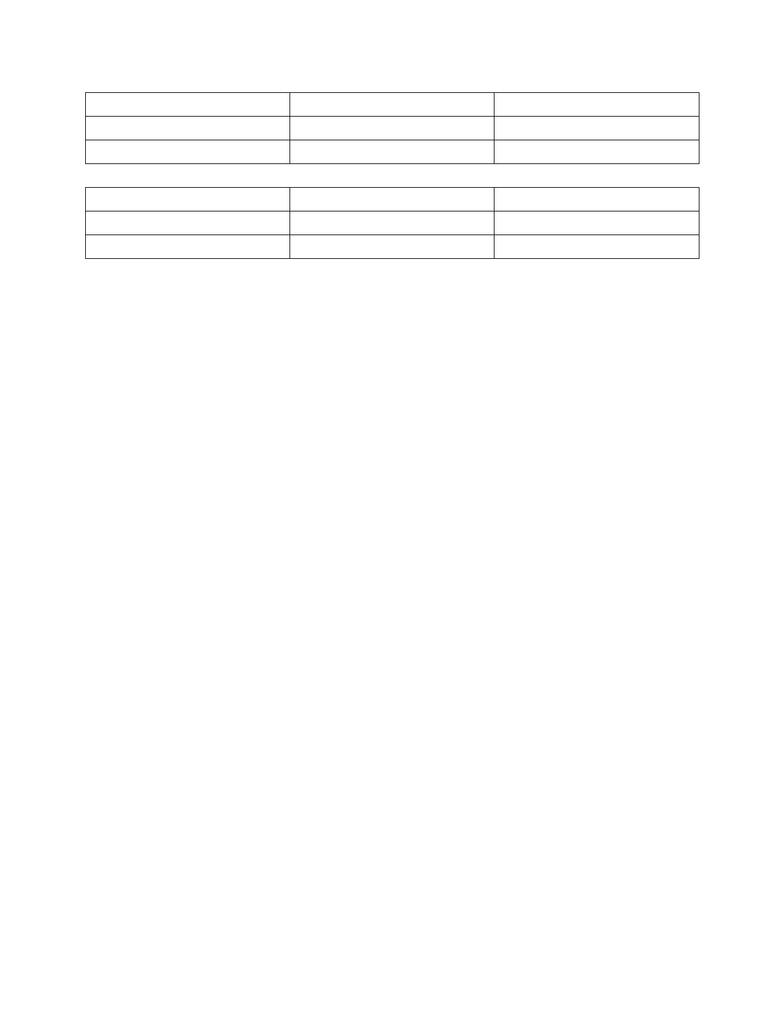Class Notes (1,100,000)
CA (650,000)
McGill (40,000)
ECON (100)
Lecture 2

# ECON 330D1 Lecture Notes - Lecture 2: Fixed Investment, Gdp Deflator, Inventory Investment

Department
Economics (Arts)
Course Code
ECON 330D1
Professor
Daniel Barczyk
Lecture
2

This preview shows pages 1-2. to view the full 6 pages of the document.Econ 330- Lecture 2 9/11/2012 10:41:00 AM
Nominal GDP is output measured at current prices
GDP- Price of Melons * Quantity of melons
+ price of grapes * Quantity of grapes
Real GDP is output measured using a constant set of prices
We need to use base year prices
E.G. chooses pries in 2010 to compute real GDP in 2010, 2011, 2012
Real GDP 2010= 2010 prices of melons * 2010 quantity of melons +
2010 price of grapes * 2010 quantity of grapes = nominal GDP as cause yrs
the same
Real GDP 2010= 2010 prices of melons * 2011 quantity of melons +
2010 price of grapes * 2011 quantity of grapes
This is where the base year is 2010
GDP deflator- nominal GDP/ Real GDP it reflects what’s happening to the
overall level of prices in the economy.
Consider the economy with a single bread if P= price of bread and Q=
quantity sold then nominal GDP = P *Q. Real GDP is quantity of bread
produced using the price of same base year = Pbase * Q. The GDP deflator
is simply P *Q/Pbase * Q= P/Pbase
Using the GDP deflator we can separate nominal GDP into a part which
measures quantities ( Real GDP) and the other measures prices (the GDP
deflator) nominal GDP = Real GDP *GDP
Real GDP = Nominal GDP/ GDP deflator
Example From PROBLEM 4 OF CHAPTER 2
YR 1

Only pages 1-2 are available for preview. Some parts have been intentionally blurred.GOOD
Q
P
Computers
20
\$1000
10000
\$1
YR 2
GOOD
Q
P
Computers
25
\$1500
12000
\$1.10
Nominal GDP 1= 20 *1000 + 10000 * 1= \$30,000
Nominal GDP 2= 25 *1500 +12000 *1.10= \$50,700
Growth = 50,700-30,000/30,000 * 100= 69%
B, Calculate GDP using year 1 as the base year
RGDP1/1 = nominal GDP1 = 30,000
RGDP ½= 25 * 1000 +12,000 *1= 37,000
Growth = 23%
C, Use Year 2 as base year
RGDP2/1= 20 *1500 +10,000 *1.10= 41,000
RGDP 2/2= 50,700
Growth = 23.65 %
D, Calculate real GDP using the chain weighted method
Gc= G1 * G2= 1.235
G1= 1.233 G2= 1.2365
Gc= 23.5
Compute real GDP for yrs 1 and 2
We cam arbitrary designate year 1 as the base year
In this case real GDP in year 1 is the same as nominal GDP
Real GDP in year 2 is equal to 1.235 * 30,000= 37,050
Designate year two as base year
Then real GDP in year 2 equals nominal GDP while real GDP in year 1 is
equal to 50,700/1.235= 41,052